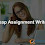In this article I am going explain how to create Shapes using Trigonometry with jQuery. JavaScript supports 3 trigonometric functions. This idea might help you if you want place elements on particular shape.
Math.sin(angle)
Math.cos(angle)

We need CSS and jQuery function to create pixels.

#### CSS :

```#circle b {
position:absolute;
color:rgb(91,111,135);
}

#circle {
position:relative;
margin:auto;
width:400px;
height:400px;
vertical-align:center;
}
```

#### putpixel function :

```function putpixel(mx,my) {
\$("#circle").append("<b style='left:"+mx+"px;top:"+my+"px'>.</b>");
}

```

### Circle :

X = X -coordinate
Y = Y -coordinate
R = Radius of the Circle
X = R cosθ
Y = R sinθ

#### jQuery :

xc, yc : Center of the Cicle
i : Angle
```var xc=200,yc=200;
var i=360,r=200;
while(i>0)
{
putpixel(xc + r*Math.cos(i),yc+ r*Math.sin(i));
i = i - 0.1;
}
```

### Ellipse :

X = X -coordinate
Y = Y -coordinate
Ra, Rb = One-half of the Ellipse's major and minor axes
X = Ra cosθ
Y = Rb sinθ

#### jQuery :

xc, yc : Center of the Cicle
i : Angle
ra, rb : One-half of the Ellipse's major and minor axes
```var xc=200,yc=200;
var i=360,ra=200, rb=100;
while(i>0)
{
putpixel(xc + ra*Math.cos(i),yc+ rb*Math.sin(i));
i = i - 0.1;
}

```

### Random shape :

X = X -coordinate
Y = Y -coordinate
a, b  = Random variables
X = a ( 1 / cosθ )
Y = b ( 1 / sinθ )

#### jQuery :

xc, yc : Center
i : Angle
a = Random variables
```var xc=200,yc=200;
var i=360,a=200, b=100;
while(i>0)
{
putpixel(xc + a*(1/Math.cos(i)),yc+ b*(1/Math.sin(i)));
i = i - 0.1;
}

```

1.I love this. It is soo informative. Are you also searching for Swedish assignment writing help we are the best solution for you. We are best known for delivering the best services to students without having to break the bank

2.Your blogs are great.Are you also searching for nursing pico writing help? we are the best solution for you. We are best known for delivering nursing writing services to students without having to break the bank.

3.This comment has been removed by the author.

## BlogrollSrinivas Dasari find me on facebook follow me on twitter